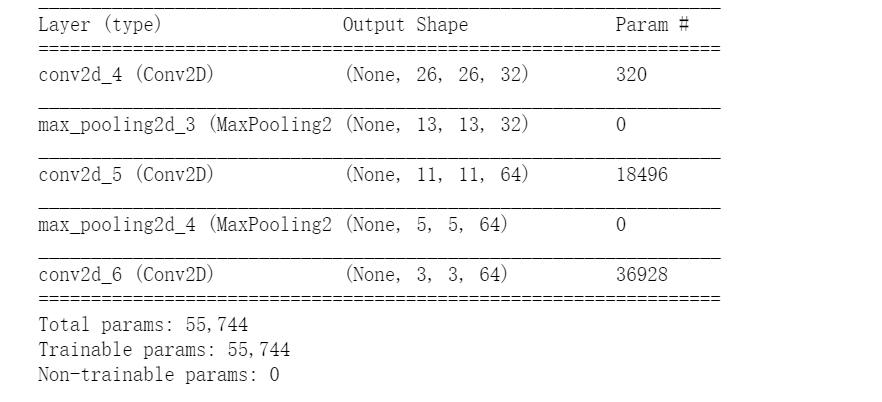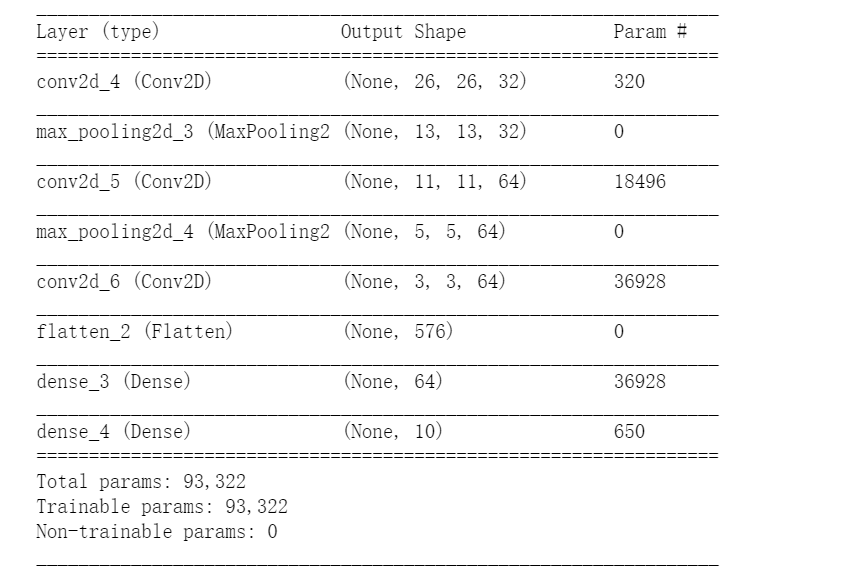# 卷积神经网络简介

from keras import layers
from keras import models

model = models.Sequential()
model.add(layers.Conv2D(32, (3, 3), activation='relu', input_shape=(28, 28, 1)))
model.add(layers.Conv2D(64, (3, 3), activation='relu'))

model.summary()model.add(layers.Flatten())
model.add(layers.Dense(10, activation='softmax'))

model.summary()from keras.datasets import mnist
from keras.utils import to_categorical

(train_images, train_labels), (test_images, test_labels) = mnist.load_data()

train_images = train_images.reshape((60000, 28, 28, 1))
train_images = train_images.astype('float32') / 255

test_images = test_images.reshape((10000, 28, 28, 1))
test_images = test_images.astype('float32') / 255

train_labels = to_categorical(train_labels)
test_labels = to_categorical(test_labels)

model.compile(optimizer='rmsprop',
loss='categorical_crossentropy',
metrics=['accuracy'])
model.fit(train_images, train_labels, epochs=5, batch_size=64)

Epoch 1/5
60000/60000 [==============================] - 10s 172us/step - loss: 0.1694 - acc: 0.9472
Epoch 2/5
60000/60000 [==============================] - 6s 107us/step - loss: 0.0477 - acc: 0.9854
Epoch 3/5
60000/60000 [==============================] - 6s 108us/step - loss: 0.0330 - acc: 0.9897
Epoch 4/5
60000/60000 [==============================] - 6s 105us/step - loss: 0.0246 - acc: 0.9929
Epoch 5/5
60000/60000 [==============================] - 6s 107us/step - loss: 0.0190 - acc: 0.9943

test_loss, test_acc = model.evaluate(test_images, test_labels)

print（test_acc）

©️2019 CSDN 皮肤主题: 技术工厂 设计师: CSDN官方博客